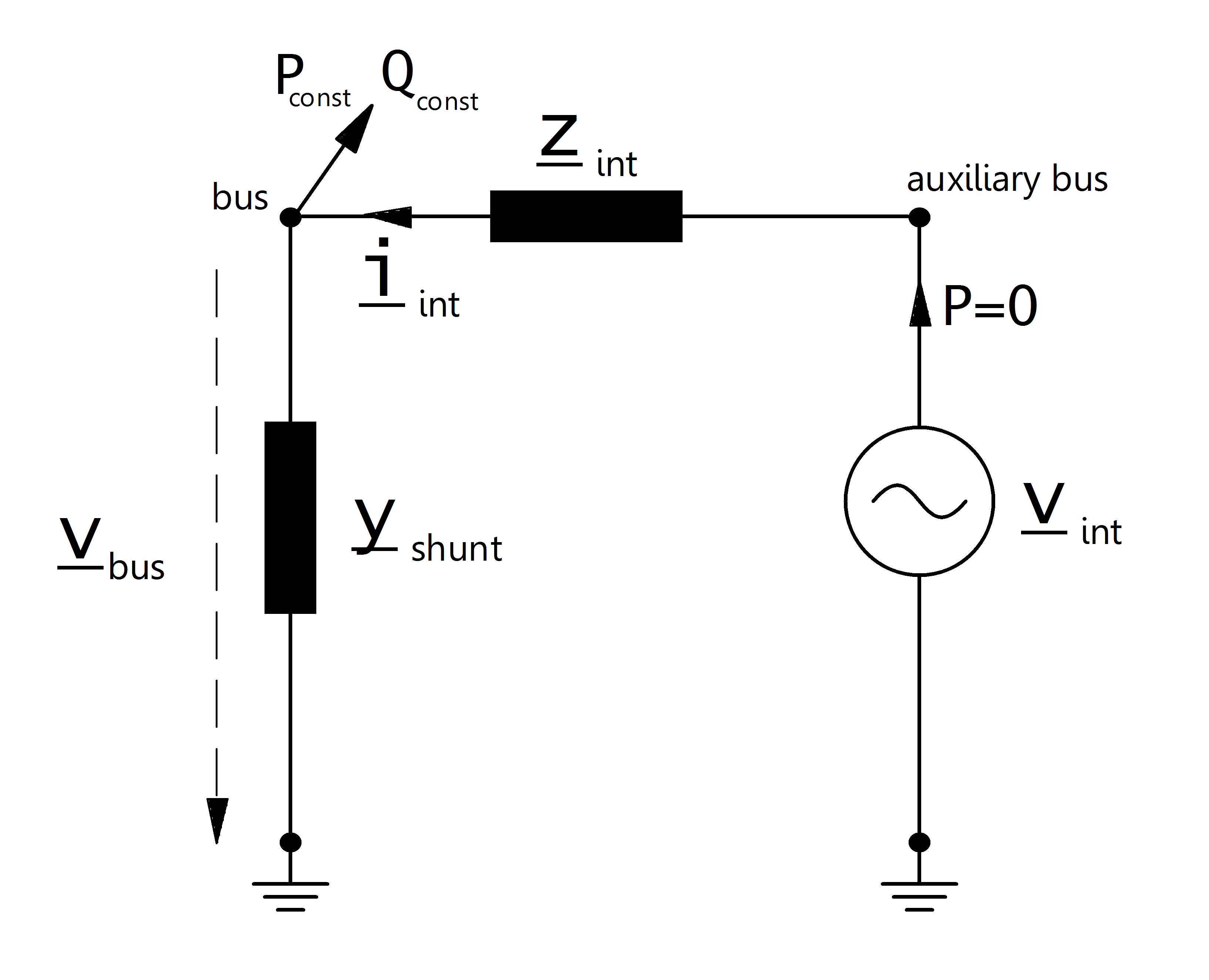# Extended Ward

## Create Function

pandapower.create.create_xward(net, bus, ps_mw, qs_mvar, pz_mw, qz_mvar, r_ohm, x_ohm, vm_pu, in_service=True, name=None, index=None, slack_weight=0.0, **kwargs)

Creates an extended ward equivalent.

A ward equivalent is a combination of an impedance load, a PQ load and as voltage source with an internal impedance.

INPUT:

net - The pandapower net within the impedance should be created

bus (int) - bus of the ward equivalent

ps_mw (float) - active power of the PQ load

qs_mvar (float) - reactive power of the PQ load

pz_mw (float) - active power of the impedance load in MW at 1.pu voltage

qz_mvar (float) - reactive power of the impedance load in MVar at 1.pu voltage

r_ohm (float) - internal resistance of the voltage source

x_ohm (float) - internal reactance of the voltage source

vm_pu (float) - voltage magnitude at the additional PV-node

slack_weight (float, default 1.0) - Contribution factor for distributed slack power

flow calculation (active power balancing)

OUTPUT:

xward id

## Input Parameters

net.xward

 Parameter Datatype Value Range Explanation name string name of the extended ward equivalent bus* integer index of connected bus ps_mw* float constant active power demand [MW] qs_mvar* float constant reactive power demand [MVar] pz_mw* float constant impedance active power demand at 1.0 pu [MW] qz_mvar* float constant impedance reactive power demand at 1.0 pu [MVar] r_ohm* float $$>$$ 0 internal resistance of the voltage source [ohm] x_ohm* float $$>$$ 0 internal reactance of the voltage source [ohm] vm_pu* float $$>$$ 0 voltage source set point [p.u] in_service* boolean True / False specifies if the extended ward equivalent is in service.

*necessary for executing a power flow calculation.

## Electric Model

The extended ward equivalent is a ward equivalent: with additional PV-node with an internal resistance.The constant apparent power is given by:

\begin{align*} P_{const} &= ps\_mw\\ Q_{const} &= qs\_mvar\\ \end{align*}

The shunt admittance part of the extended ward equivalent is calculated as described here:

\begin{align*} \underline{y}_{shunt} &= \frac{pz\_mw + j \cdot qz\_mvar}{S_{N}} \end{align*}

The internal resistance is defined as:

\begin{align*} \underline{z}_{int} &= r\_pu + j \cdot x\_pu \end{align*}

The internal voltage source is modelled as a PV-node (generator) with:

\begin{align*} p\_mw &= 0 \\ vm\_pu &= vm\_pu \end{align*}

## Result Parameters

net.res_xward

 Parameter Datatype Explanation p_mw float active power demand of the ward equivalent [MW] q_mvar float reactive power demand of the ward equivalent [MVar] vm_pu float voltage at the ward bus [p.u]
\begin{align*} vm\_pu &= v_{bus} \\ p\_mw &= P_{const} + Re(\frac{\underline{V}_{bus}^2}{\underline{Y}_{shunt}}) + Re(\underline{I}_{int} \cdot \underline{V}_{bus}) \\ q_mvar &= Q_{const} + Im(\frac{\underline{V}_{bus}^2}{\underline{Y}_{shunt}} + Im(\underline{I}_{int} \cdot \underline{V}_{bus}) ) \end{align*}